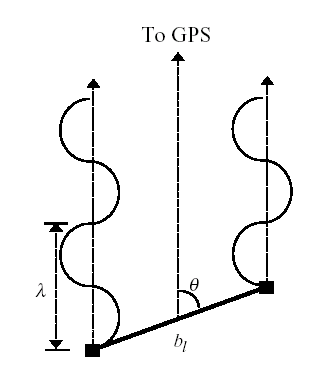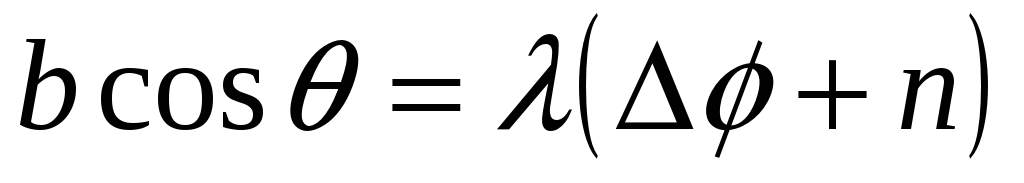# Spacecraft Attitude Determination Using Global Positioning Satellite Signals

 Spacecraft Attitude Determination Using Global Positioning Satellite Signals C1C Andrea M. Johnson United States Air Force Academy Abstract Unmanned spacecraft are key to gathering information about the universe in inexpensive, low-risk ways. Critical to the success of remote sensing satellites is accurate attitude determination and control. One method of attitude determination that is relatively inexpensive, but has relatively little flight history is the use of Global Positioning System (GPS) satellites. To determine integer phase differences between GPS receivers, this paper will use a variation of integer search: an arrangement of three receivers in a line and two GPS satellites in view. The next step to attitude determination is the minimization of the loss function. Several methods already explored include linear least-squares, and the Attitude-Lean-Loping-Estimator using GPS Recursive Operations (ALLEGRO) algorithm. The Gauss-Newton and Gauss-Newton-Levenberg-Marquardt methods are used and compared to these two methods. The Gauss-Newton and Gauss-Newton-Levenberg-Marquardt both prove to be inferior to these methods in accuracy and computational demand. The reasons for this include the simple receiver orientation, the use of only two GPS satellites, errors created by attempting to minimize a matrix using an algorithm designed for vectors, and the use of a constant in the Gauss-Newton-Levenberg-Marquardt method to avoid singularities. It is superior to these methods in the fact that it requires a single set of fractional phase differences between receivers to determine the spacecraft attitude and requires no convergence time. Introduction Attitude determination using GPS signals involves using multiple receivers on a satellite and determining total phase differences in a signal from one receiver to another. The line connecting these two receivers is called the baseline. Since the satellite is thousands of kilometers away from the GPS satellite, it can be assumed that the signals reaching the receivers are parallel as shown in figure 1 (Application of Vectorized Attitude Determination Using Global Positioning Signals).Figure 1: Baseline orientation with respect to GPS signal (Application of Vectorized Attitude Determination Using Global Positioning Signals) The relationship between the angle at which the baseline is oriented and the phase difference in signal between the two receivers can be expressed as(1) Download 1.81 Mb.Share with your friends: